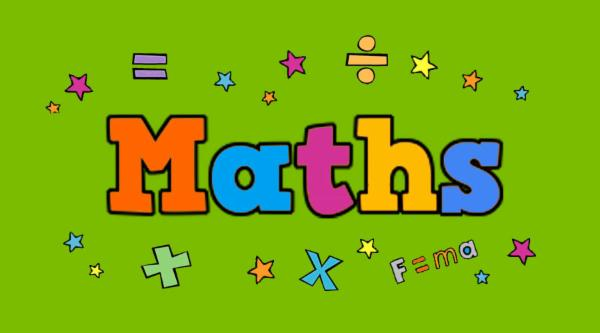## CBSE 8th Grade MATHS

Central Board of Secondary Education

Animated Video CBSE Syllabus 8th Grade Maths

Instructor: Launch discount 45% off

Language: English

Validity Period: 350 days

Max Viewing Hours: 100 Hours

₹6666 44% OFF

## ₹3690

10 Credits INR as Cashback

CBSE - VIII MATHS

CHAP 1 : RATIONAL NUMBERS : Introduction on Rational Numbers. Properties of Rational Numbers, Binary Operations, Closure Property, Commutative Property, Associative Property, Rational Number Egs in all Properties, Eg:1, 2, Role of Zero, Role of One, Negative of Numbers, Ex:1.1.Representation of Rational Numbers on the Number Line, Rational Numbers Between Two Rational Numbers, Eg:6, 8, Ex:1.2.

CHAP 2 : LINEAR EQUATION IN ONE VARIABLE : Introduction Linear Equations in One Variable, Eg:2, 3, Ex:2.1.Eg:10, 11, Ex:2.2, Solving Equations Variables on Both Sides of Equation, Linear Equations, Egs.

CHAP 3 : UNDERSTANDING QUADRILATERALS : Intro on Understanding Quadrilaterals, Polygons, Classification Of Polygons, Diagonal, Concave and Convex Polygons, Regular and Irregular Polygons, Angle Sum Property, Measure of Exterior angle of Polygon, Types of Quadrilaterals, Trapezium, Kite, Parallelogram, Elements of Parallelogram, Diagnosis of Parallelogram, Rhombus, Rectangle, Square.

CHAP 4 : PRACTICAL GEOMETRY : Introduction on Practical Geometry, Construction of Quadrilaterals, Properties of Construction of Quadrilateral, Four Sides and One Diagonal, Three Side and Two Diagonals, Two Adjacent Sides and Three Angles, Three Sides and Two Included Angles, Special Quadrilaterals.

CHAP 5 : DATA HANDLING : Introduction on Data Handling, Pictograph, Bar Graph, Double Bar Graph, Organising Data, Grouping Data, Bars with Differences, Histogram, Pie Charts, Chances and Probability, Outcomes and Events.

CHAP 6 : SQUARES AND SQUARE ROOTS : Introduction on Square roots, Property of Square Numbers, Square Roots Basics and some Egs Finding Square Root, Prime Factorisation, By Division,Square Roots By Decimal Numbers. Estimation of Square Roots.

CHAP 7 : CUBES AND CUBE ROOTS : Introduction, Perfect Cubes, Properties of Cube numbers, Cube Of Negative Integers, Cube Roots, Prime Factorisation, Negative Number, Product of Integer, Rational Number.

CHAP 8 : COMPARING QUANTITIES : Intro on Recalling Ratios, Percentage, Finding Increase and Decrease, Discounts, Estimation in Percentages, Cost Price, Selling Price, Sales Tax, Intro on Compound Interest, Formula For Compound Interest, Rate Per Annual Compounding Half Yearly.

CHAP 9 : ALGEBRAIC EXPRESSION AND IDENTITIES : Introduction Algebra Expressions, Terms, Factors, Coefficients,, Monomial, Binomial, Polynomial, Like and Unlike Terms, Common Egs , Addition and Subtraction Of Algebraic Expressions, Multiplication Of Algebraic Expressions, Multiply Monomials, Multiply By Three or More Monomials, Multiply Monomial By Polynomial, Multiply Trinomial By Binomial. Multiply Polynomial By Polynomial, Common Egs On Multiply Polynomial By Polynomial, Identity, Standard Identities. Common Egs.Applying Identities, Common Egs.

CHAP 10 : VISUALISING SOLID SHAPES : Intro on Visualising Solid Shapes, Views Of 3D Shapes, Mapping Space Around us. Intro on Faces, Edges, Vertices, Polyhedron, Convex Polyhedrons, Regular Convex Polyhedron, Prisms, Pyramid.

CHAP 11 : MENSURATION : Intro on Mensuration, Perimeter, Area,Area Of Trapezium, Area of General and Special Quadrilateral, Eg:1, 2, Area of Polygon,  Intro on Solid Shapes, Surface Area Of Cube, Cuboid, Surface area of Cylinder, Volume of Cube, Cuboid, Cylinder. Volume Of Capacity.

CHAP 12 : EXPONENTS AND POWERS : Intro on Exponents and Power, Negative Exponents, Laws of Exponents, Use of Exponents to Express Numbers in Standard Form, Comparing Very Large and Very Small Numbers.

CHAP 13 ; DIRECT AND INVERSE PROPORTION : Introduction, Direct Proportions, Direct Proportions Examples, Intro on Inverse Proportions, Inverse Proportions Examples.

CHAP 14 : FACTORISATION : Introduction Factorisation, Factors of Natural Numbers, Algebraic Numbers, Methods of Factorisation, Factorisation By Grouping Terms, Factorisation Using Identities,Factorisation of Quadratic Expressions, Division by Algebraic Expression,  Overall Examples.

CHAP 15 : INTRODUCTION TO GRAPHS : Introduction, Bar Graph, Pie Graph, Line Graph, Coordinates, Examples on all the Topics.

CHAP 16 : PLAYING WITH NUMBERS : Introduction, Numbers in General Form, Games with Numbers, Reversing the Digits, Letters for Digits, Tests of Divisibility.

NOTE : Few more Exercises and Examples from the textbook will be uploaded soon.

JARGONS : Egs = Examples   Ex = Exercise

Reviews
Other CoursesLaunch your Graphy